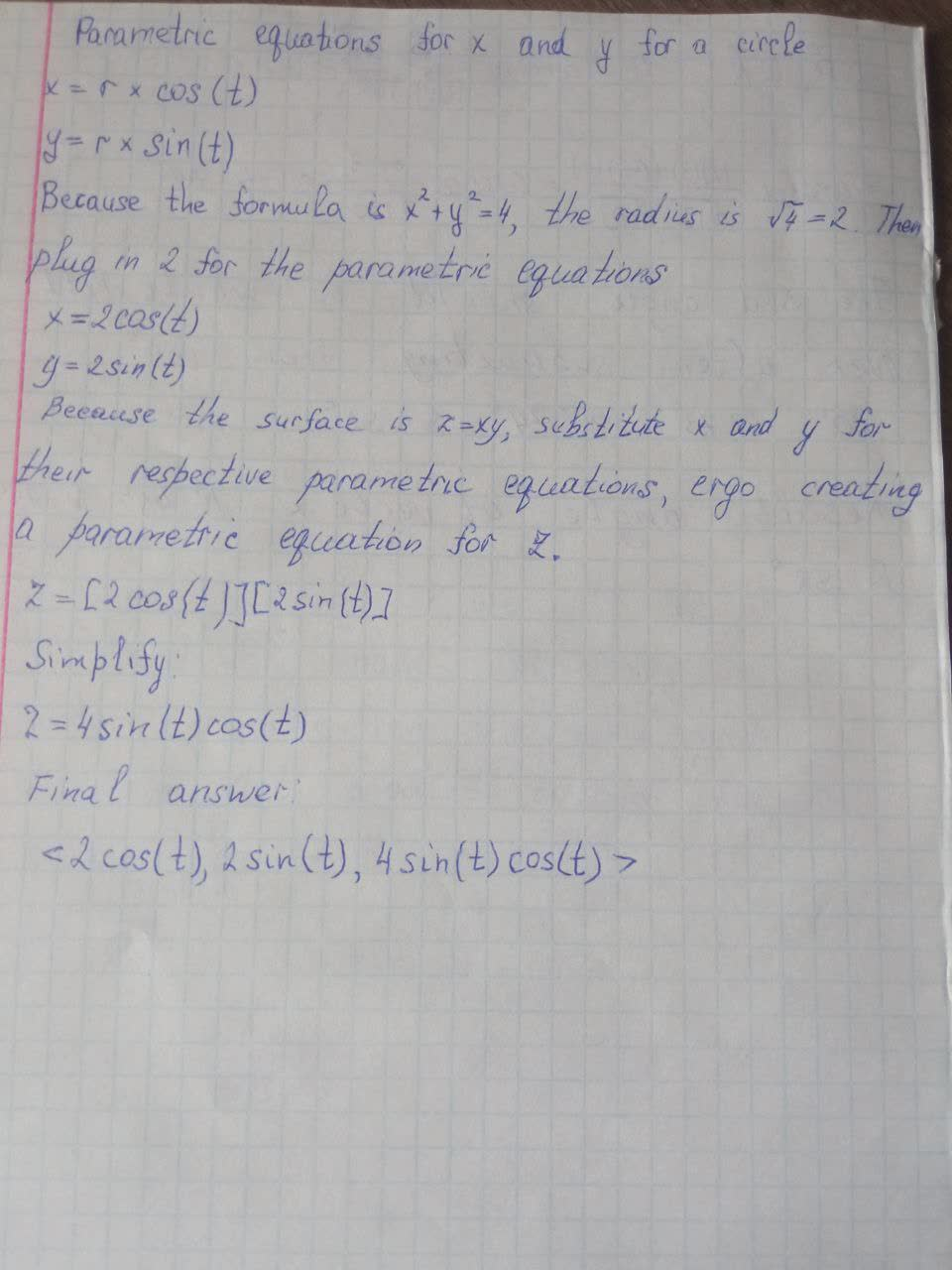Question# Find a vector function that represents the curve of intersection of the two surfaces. The cylinder x^2+y^2=4 and the surface z=xy

Vectors
ANSWEREDFind a vector function that represents the curve of intersection of the two surfaces. The cylinder $$x^2+y^2=4$$ and the surface z=xy